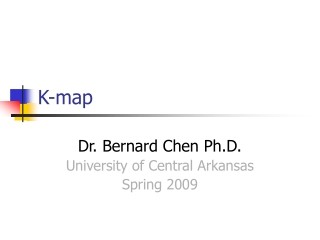DownloadDownload PresentationK-map

# K-map

Download Presentation## K-map

- - - - - - - - - - - - - - - - - - - - - - - - - - - E N D - - - - - - - - - - - - - - - - - - - - - - - - - - -
##### Presentation Transcript

1. K-map Dr. Bernard Chen Ph.D. University of Central Arkansas Spring 2009

2. Karnaugh Maps (K-maps) • Karnaugh maps -- A tool for representing Boolean functions of up to six variables. • K-maps are tables of rows and columns with entries represent 1`s or 0`s of SOP and POS representations.

3. Karnaugh Maps (K-maps) • An n-variable K-map has 2n cells with each cell corresponding to an n-variable truth table value. • K-map cells are labeled with the corresponding truth-table row. • K-map cells are arranged such that adjacent cells correspond to truth rows that differ in only one bit position (logical adjacency).

4. Karnaugh Maps (K-maps) • If miis a minterm of f, then place a 1 in cell i of the K-map. • If Mi is a maxterm of f, then place a 0 in cell i. • If diis a don’t care of f, then place a d or x in cell i.

5. Examples Two variable K-map f(A,B)=m(0,1,3)=A`B`+A`B+AB A 0 1 B 0 1

6. Three variable map f(A,B,C) = m(0,3,5)= A`B`C`+A`BC+AB`C A`B`C`

7. Maxterm example f(A,B,C) = M(1,2,4,6,7) =(A+B+C`)(A+B`+C)(A`+B+C) )(A`+B`+C) (A`+B`+C`) Note that the complements are (0,3,5) which are the minterms of the previous example (A+B) (A+B`) (A`+B`) (A`+B) C C`

8. Four variable example(a) Minterm form. (b) Maxterm form. f(a,b,Q,G) = m(0,3,5,7,10,11,12,13,14,15) = M(1,2,4,6,8,9)

9. Simplification of Boolean FunctionsUsing K-maps K-map cells that are physically adjacent are also logically adjacent. Also, cells on an edge of a K-map are logically adjacent to cells on the opposite edge of the map. If two logically adjacent cells both contain logical 1s, the two cells can be combined to eliminate the variable that has value 1 in one cell’s label and value 0 in the other.

10. Simplification Guidelines for K-maps Always combine as many cells in a group as possible. This will result in the fewest number of literals in the term that represents the group. Make as few groupings as possible to cover all minterms. This will result in the fewest product terms. Always begin with the largest group, which means if you can find eight members group is better than two four groups and one four group is better than pair of two-group.

11. Example Simplify f= A`BC`+ ABC`+ ABC using;(a) Sum of minterms. (b) Maxterms. Each cell of an n-variable K-map has n logically adjacent cells. F`= B`+A`C F = B(A+C`) a- f(A,B,C) = AB + BC b- f(A,B,C) = B(A + C)

12. Example Simplify f(A,B,C,D) = m(2,3,4,5,7,8,10,13,15)

13. Example Multiple selections f(A,B,C,D) = m(2,3,4,5,7,8,10,13,15) c produces less terms than a

14. Example Redundant selectionsf(A,B,C,D) = m(0,5,7,8,10,12,14,15)

15. Example

16. Example

17. Example

18. f(A,B,C,D) = m(1,2,4,6,9)

19. Different styles of drawing mapsf(A,B,C) = m(1,2,3,6) = AC + BC BC 00 01 11 10 A 1 1 1 0 1 1 C 0 1 AB 00 01 11 10

20. Don’t-care condition • Minterms that may produce either 0 or 1 for the function. • They are marked with an ´ in the K-map. • This happens, for example, when we don’t input certain minterms to the Boolean function. • These don’t-care conditions can be used to provide further simplification of the algebraic expression. • (Example) F = A`B`C`+A`BC` + ABC` • d=A`B`C +A`BC + AB`C • F = A` + BC`

21. Five variable K-mapsUse Two Four-variable K-Maps f(a,b,c,d,e) = m(0,5,7,13,15,16,21,23,29,31) a`=0 map a=1 map 1 1 1 1 1 1 1 1 1 1

22. F=(a+a`)ce + (a+a`)b`c`d`e`=ce + b`c`d`e` a`=0 map a=1 map 1 1 1 1 1 1 1 1 1 1 F1=a`b`c`d`e` + a`ce, F2=ace + ab`c`d`e` f(a,b,c,d,e) = f1+f2